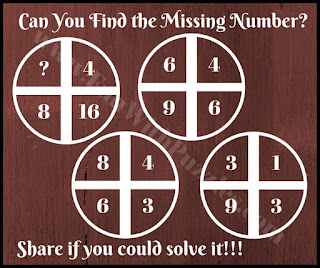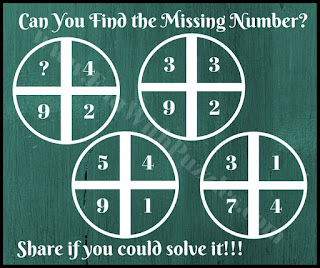Mathematics offers a wide range of brain teasers. Using only arithmetic operations, many mind-blowing maths questions can be created. Here on the website, one can find a wide range of Maths Questions which range from finding the missing number in the series to solving Maths Equations with shapes and symbols.
Also, there are many different types of puzzles that are construed using different Trigonometrical shapes. Today's Mind Blowing Questions are based on the Circle shape which is the extension of the similar questions which are posted with the title "Mathematical Cross Circle Fun Brain Teasers".
In each of these Mind-blowing questions, four numbers are given in a circle. These numbers are related to each other. Three circles contain all four numbers which will help you to establish the relationship between these numbers. However, in one of the Circle one number is missing. You have to find the relationship between these numbers and then find the value of the missing number which will replace the question mark.
Are you ready to solve these mind-blowing questions? These questions are very tough and the first four answers are provided for these puzzles for the last answer we are as we are looking forward to the visitors here solving these and posting their comments with the explanation. Looking forward to your comments :)Mind-Blowing Question-1Mind-Blowing Question-2Mind-Blowing Question-3Mind-Blowing Question-4Mind-Blowing Question-5

Some of these Circle Questions were really mind-blowing? How many of these were you able to solve without looking at the answers. If you are able to solve more than two of these tough Maths Circle Puzzles then you have a very good mind and you should try some of these below-mentioned brain teasers to challenge your brain

## The answer to Mind-Blowing Maths Circle Questions

To understand the logic used in these Mathematical patterns, let's first denote the numbers given inside the Circle with letters. Reading from left to right and up to down, let's mark the numbers with A, B, C, and D respectively. These notations will help the explanations of these tough mind-blowing Maths questions.

1)
The logic used in this Mathematical pattern is A x A + B = C x 10 + D

2)